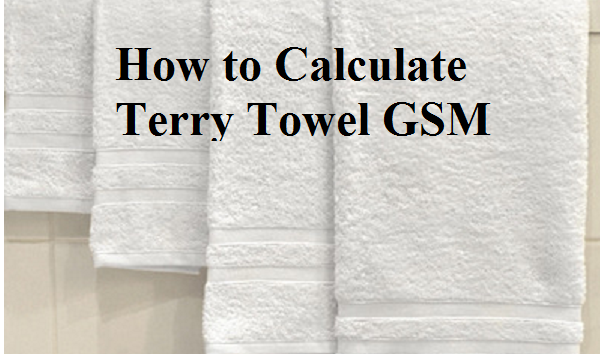# How to Calculate GSM of a Terry Towel?

Here GSM stands for weight in Grams per Square Meter. This means the weight of one square meter of the towel is expressed as towel GSM.Like other textile fabrics, GSM of the terry towel is calculated using the following method.

First method:

Cut a test specimen from the towel. Normally, no towel comes 1 meter in width. So, cut a smaller size specimen in a square shape. Let's cut 20 cm X 20 cm from the towel. Weight this specimen.

Let's us assume the weight is 20 grams for 0.04 square meter towel specimen.
Now convert the weight for a towel dimension of 1 square meter.

GSM would be (20/0.04) =500 grams per square meter

Second method:

Let's assume that you have a towel sized 50 cm X 80 cm and it weighs 240 grams. What would be GSM of this towel?

Measure the towel weight per square meter (GSM) by using the following formula.Calculation

Sample GSM
= (240 * 10000)/(50 * 80) grams/square meter
= (240 /4) = 600 grams/m2